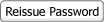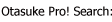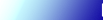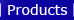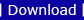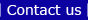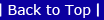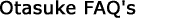CompatibilityFor Integer Array, when accessing a bitAfter conversion from GP-Pro/PBIII to GP-Pro EX, two differences are made as described below.

1) Converted to a description with the number of elements specified.

Only the description changes. No work is necessary after conversion and the operation has no problem.

 EX) For an array of integer variables, when the 250th bit is specified, Specified on GP-PRO/PBIII C-Package : IntegerArray.XDisplay on GP-Pro EX : IntegerArray.X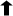The number of elements

For GP-PRO/PBIII, a bit can be directly specified without the number of elements specified. For GP-Pro EX, the same type of description is not retained. After conversion to GP-Pro EX, the description is converted to one with the number of elements automatically specified.

An integer variable is 32-bit.
250 divided by 32 is "7 and the remainder is 26".

The 250th bit of the integer variable of [Integer Array] is recognized as the 26th bit in the element 7 of [Integer Array] and it's displayed as Integer Array.X on GP-Pro EX.

2) If a bit position is indirectly specified with a variable, an error will occur.

EX) IntegerArray.X[IntegerVariableA]A bit position is specified with a variable

This type of variables cannot be converted for GP-Pro EX and an error occurs.
Make some changes following the steps below.

Procedure
1. Delete the integer variable that is a source of the error (EX: [Integer Array.X) from the logic program on GP-PRO/PBIII.
2. Change the logic program on GP-PRO/PBIII as shown below.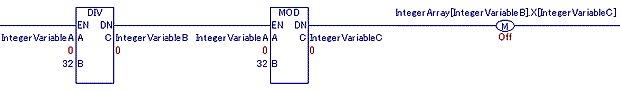Change the type to the one that specifies the number of elements like [Integer Array.X] is changed to [Integer Array.X].
Divide the variable for bit set, [Integer VariableA] by 32 to calculate the number of elements and specify it with a variable.
And specify [the remainder] that shows a bit position with a variable.

3. Perform [Convert] on GP-Pro EX.

■How to change a logic program

1. Using the DIV (division operation) instruction, divide [Integer VariableA] by 32 and assign the result to the newly created [Integer VariableB]. Use the value assigned to [Integer VariableB] as Element Number Set of [Integer Array] variables.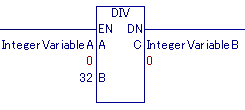2. The [remainder] of the calculation result after dividing [Integer VariableA] by 32 is Bit Position Set in an element.
Using the MOD (remainder operation) instruction, calculate a remainder and assign the result to the newly created [Integer VariableC]. Use the value assigned to [Integer VariableC] as Bit Set.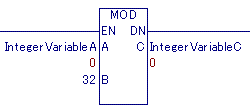3. Use Element Number Set ([Integer VariableB]) and Bit Position Set ([Integer VariableC]) that have been created in 1 and 2 instead of [Integer Array.X[Integer VariableA]] and output them with the retained and specified OUT (Out Coil) instruction.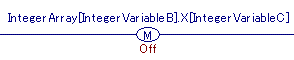[Integer Array.X[Integer VariableA]] is changed to an integer array [Integer VariableB].X[Integer VariableC] and it can be used without causing an error even after conversion to GP-Pro EX.